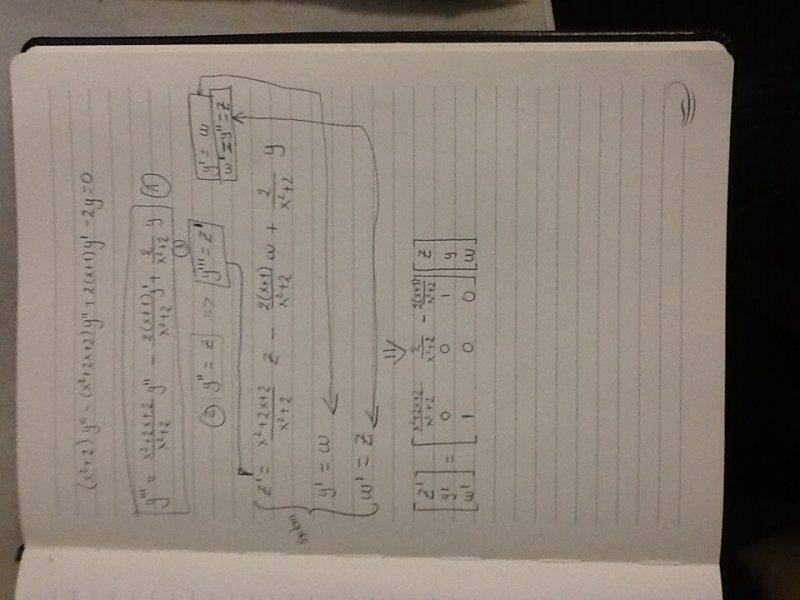# Another strange ODE

Hello again,

I've got another trouble with a new differential equation:

$(x^2+2)y'''-(x^2+2x+2)y''+2(x+1)y'-2y=0$

I did a try using matrices(file is attached), however the system to be solved is hard to compute.Do you have any idea to help me solve this out?

## Answers and Replies

Since it is probably a training exercise, the result can certainly be expressed with elementary functions. So, before using more complicated approachs, one can try to find some convenient very simple functions.
One first idea is to try exponential. The form of the coefficients in the ODE draw to check and observe that ex is a particular solution.
An other idea is to try polynomials. Trying first order polynomials is not succesfull. Then, trying second order polynomials, on the form y=x2+bx+c leads too two particular solutions : y=x2-2 and y=x2+2x. Now we have three indepêndant particular solutions. So the general solution is :
y=c1 ex + c2 (x2-2) + c3 (x2+2x)

There is a pattern to the ODE that suggests a clever substitution. How does the polynomial multiplying the third derivative differ from the polynomial multiplying the second derivative? Whats different between the polynomials multiplying the second and first derivative?